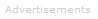# Examples of Gas Law Calculations

The relationship between the number of moles of a gas and its pressure, volume, and temperature can be calculated using the ideal gas law.### The Gas Laws

Ideal Gases
The ideal gas law can be stated in a number of ways. The equation most suited to chemistry is:

PV = nRT
P is pressure in pascals
V is volume in m3
n is number of moles
R is the gas constant = 8.314 J K-1 mol-1
T is temperature in K

The gas law equation can also be used with other units.

### Example 1

Question
A sealed jar whose volume is exactly 1 liter contains exactly 1 mole of air at 20.00 °C. Assuming the air behaves as an ideal gas, what is the pressure inside the jar in pascals? Comment on your answer.

Solve using the ideal gas equation, PV = nRT.

(a) Rearrange the equation to show P as the subject:

P = nRT / V

(b) Write down the known values with the correct SI units:

n = 1
R = 8.314 J K-1 mol-1
T = 20 °C = (20 + 273.15) K = 293.15 K
V = 1 liter = 0.001 m3

(c) Put the values into the equation P = nRT / V:

P  =  (1 × 8.314 × 293.15) / 0.001

⇒  P  =  2,437,249

⇒  P  =  2.437 × 106 Pa

Comment: The pressure is almost 24 atmospheres. The jar must be a strongly built one.

### Example 2

Question
A car tire's pressure is measured to be 200,000 Pa; its volume is 10.00 liters; and its temperature is 30.00 °C.

I) How many moles of gas are in the tire?

II) Assuming air is 80% nitrogen and 20% oxygen, what mass of air is in the tire?

I)

Use the ideal gas equation, PV = nRT.

(a) Rearrange the equation to show n as the subject:

n = PV / RT

(b) Write down the known values with the correct SI units:

P = 200,000 Pa
V = 10 liter = 0.01 m3
R = 8.314 J K-1 mol-1
T = 30 °C = (30 + 273.15) K = 303.15 K

(c) Put the values into the equation n = PV / RT:

n  =  (200,000 × 0.01) / (8.314 × 303.15)

⇒  n  =  0.7935 moles

II)

molecular mass of N2 = 2 × 14.01 = 28.02

molecular mass of O2 = 2 × 16.00 = 32.00

molecular mass of air = (80% × 28.02) + (20% × 32.00) = 28.816

mass of air = moles × molecular mass = 0.7935 × 28.816 = 22.87 g